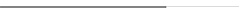## 2020年成人高考高起点理工类数学真题及答案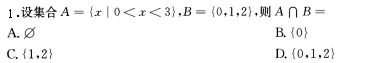2.函教y=2sinxcosx的最小正周期是（）

A.π/2   B.π   C.2π   D.4π

3.等差数列{an)中，若a1=2，a3=6，则a7=（）

A.14   B.12   C.10   D.8

4.将一颗骰子抛掷1次，则得到的点教为偶数的概率为（）

A.2/3   B.1/2   C.1/3   D.1/6

5.不等式|2x-3|＜1的解集为（）

A.{x|1<x<3}

B.{x|x<-l 或 x>2}

C.{x|1<k<2}

D.{x|2<x<3}

6-下列函数中，为偶函教的是（）

A.y=log2x   B.y=X2+X   C.y=4/x   D.y=x2

7.若tanα=3,则tan(α+π/4)=

A.2   B.1/2   C.-2   D.-4

8.642/3+log1/981=

A.8   B.10   C.12   D.14

9.曲线y=x2+l与直线y=2x的交点坐标为（ )

A.(1.2)   B.(-1，2)  C.(2，4)   D.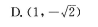10.已知正六棱锥的底面边长为3，侧棱长为5，则该六棱锥的体积为（）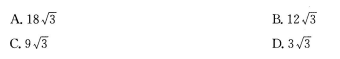11.过点（0，1)且与直线x+y+1=0垂直的直线方程为（）

A.y=x   B.y=2x+l    C.y=x+1    D.y=x-l

12.设双曲线x2/16-y2/9=1的渐近线的斜率为k，则|k|=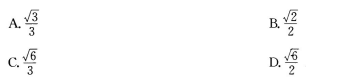14.若函数y=(αx+1)/(2x-3)的图像与其反函数的图像重合，则α=

A.-3   B.1   C.2    D.3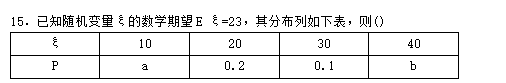A.a=0.4，b=0.3

B.a=0.3，b=0.4

C.a=0.2，b=0.5

D.a=0.5，b=0.2

16.在（l+2x)8的展开式中，22的系数为（)

A.16

B.28

C.56

D.112

17.曲线y=x3-4x+2在点（1，-1)处的切线方程为（）

A.x-y-2=0

B.x-y=0

C.x+y=0

D.27+y-2=0

18.若平面向量a=(x,1),b=(1,-2),且a//b，则x=

19.若二次函数f(x)=ax2+2x的最小值为-1/3，则a=

20.复数（2-2i）/（1+i）2的模为

22.（本小题满分12分）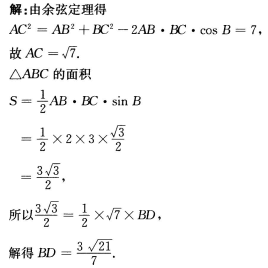23.（本小题满分12分）

（1）求{an}的通项公式；

（2）求{an}的前5项和。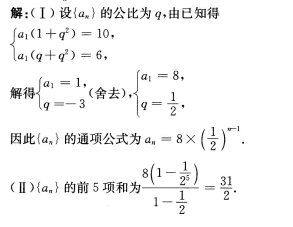24.（本小题满分12分）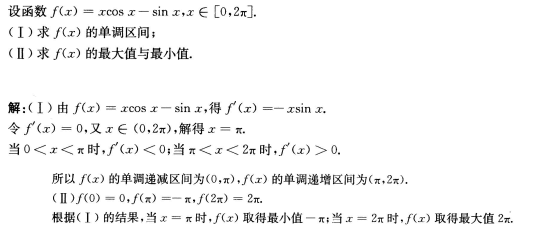25.（本小题满分13分）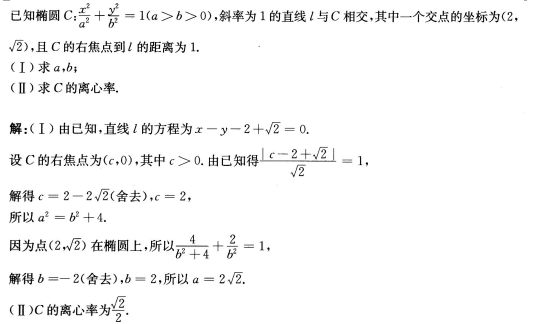## 在线课堂

### 华侨大学夜大学成人高考在线课堂### 华侨大学夜大学自学考试、网络教育在线课堂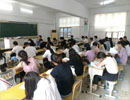### 2021年福建省职称（资格）考试在线课堂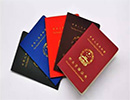2021年福建省职称（资格）考试在线课堂 [详细]